# following people

https://youtu.be/zjGXJbm8xuE

The obniz block program also understands if a person is on the left or on the right.

It makes even a robot to chase the man. let's do it.

## How to chase

If there are people, all it have to do is go straight.

But what if the person was a little to the right? You want it to move to the right.

It can chase people by "moving in the direction of people".

To do this, it need to know the position of the person (the position of the face in the camera).
And it has to change the movement of the motor based on that position

## The position of the person

Use the "Face Position" block to find out where the face is in the picture.This block gives a numerical answer as to which side the face is on.
It gets bigger as something goes to the right,
The leftmost is -100, the center is 0, and the rightmost is 100.
So, if it is a little to the right of the center, it will be 10 or 20.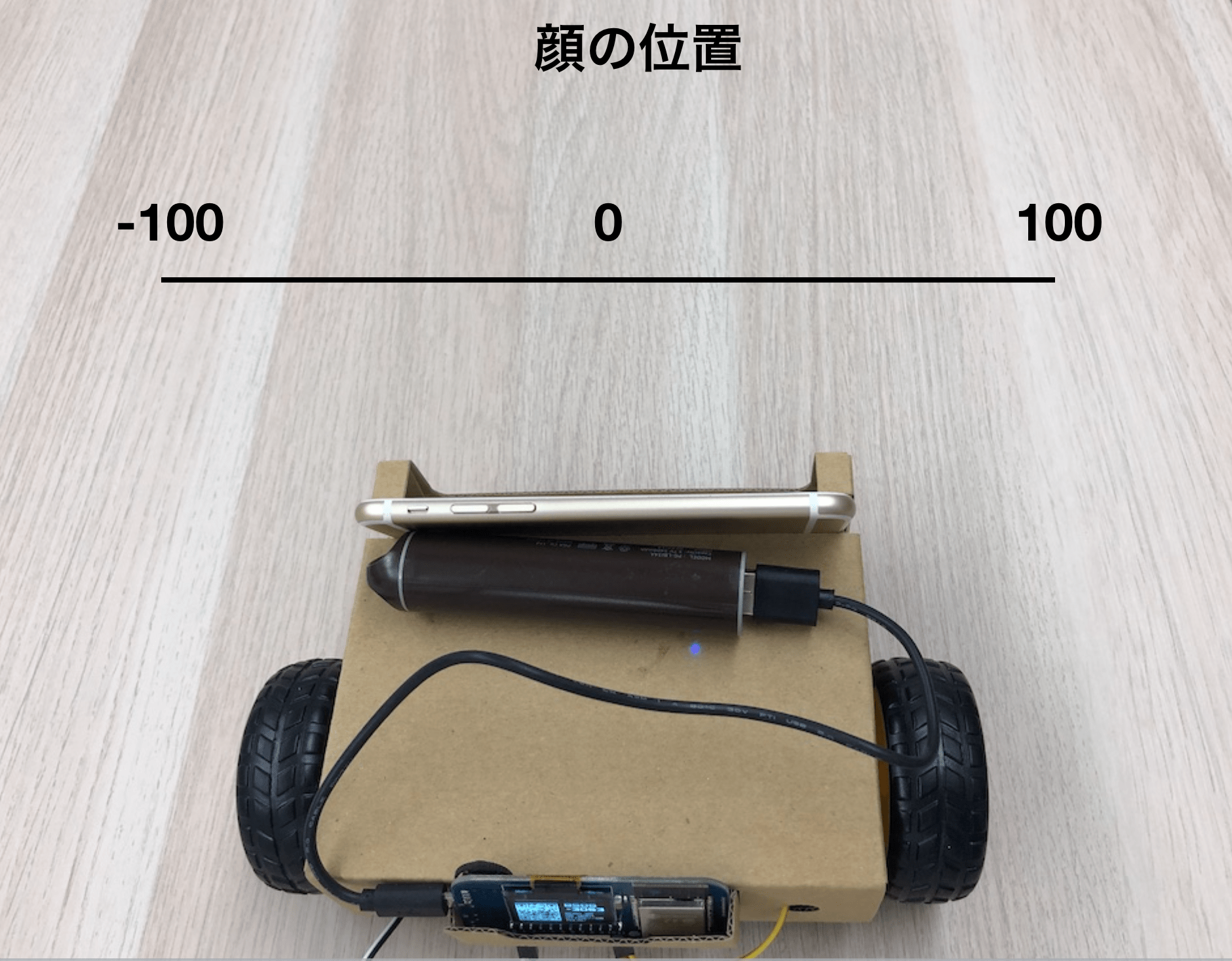Let's use this to chase people.

## How to move.

Let's divide the movement into three parts.

1. If something is on the left, go to the left
2. If something is in the middle, go straight ahead
3. If something is on the right, go to the right

It can now see the location in the "Face Position" block.
\ It is -100 to 100, we will divide it into three.
\ For -100 to -50, the face is on the far left, so set it to "go left".
\ For -50 to 50, there is a face in the middle, so go straight.
50 to 100 will be "go right".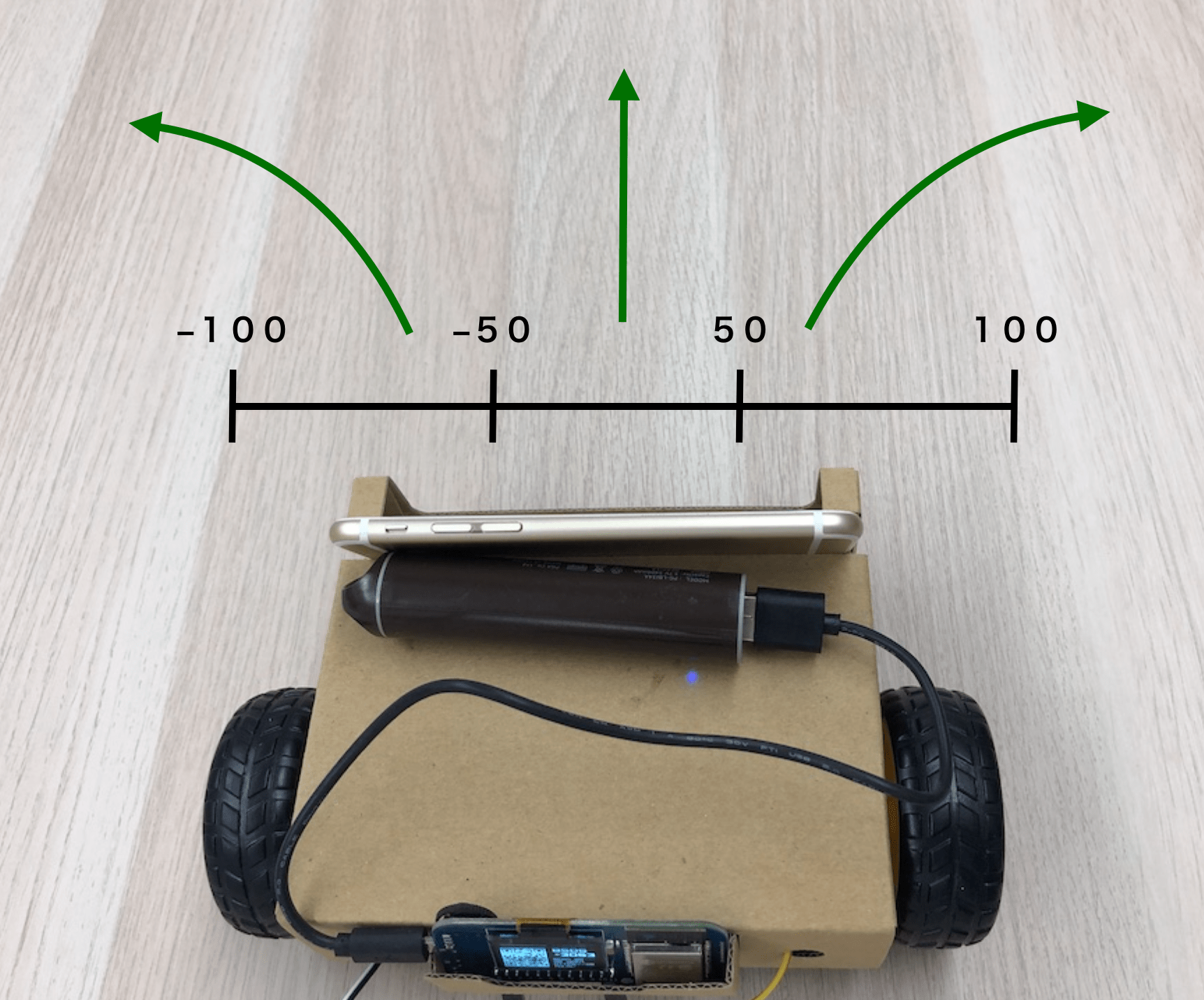## Block program

First, check with a loop to see if the face is visible.
If obniz can't see it, we want it to stop, so we'll put a block to stop it.
Then it will be like this.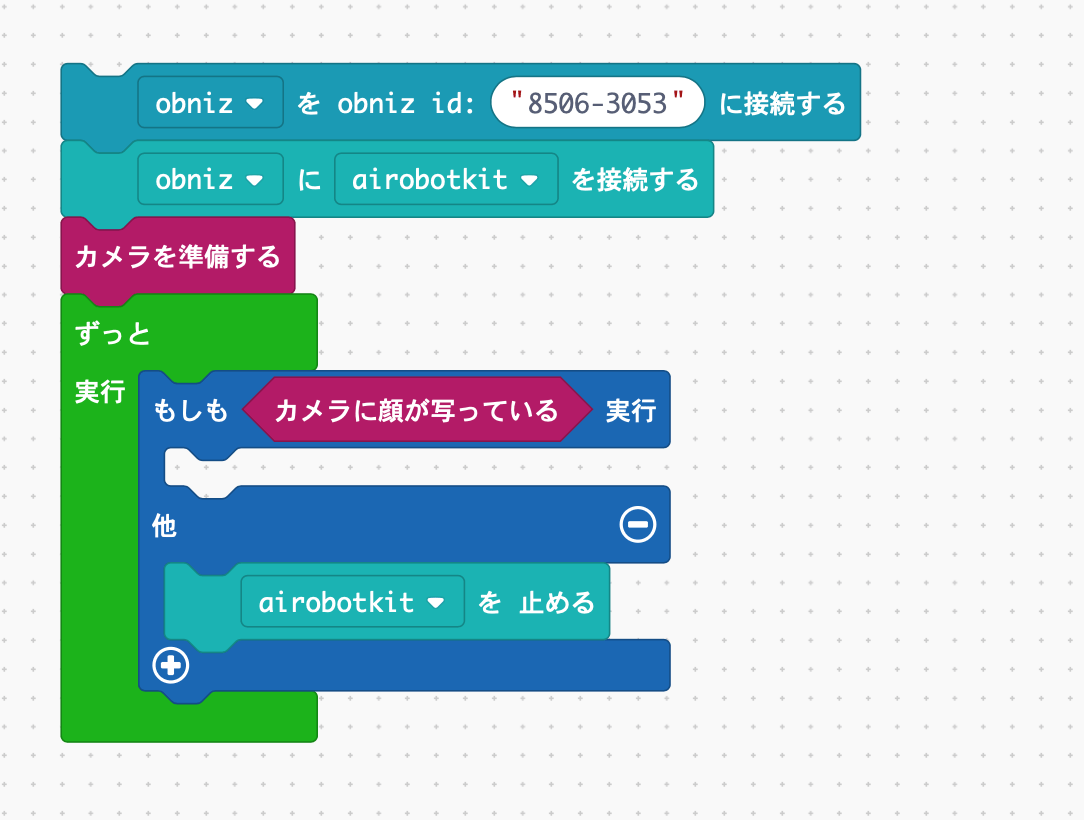The problem is from here.
We want to change the movement depending on the position of the face in this "If the face is in the picture".

First, let's make a case where the face is on the right side.
Bring a "If" block.And if you bring a block to compare the numbers in "logic" to the condition.It's like this.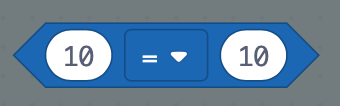This compares two numbers. 10 = 10 is correct, so the block inside what happens is executed.

Put "Position on face" here.
Then, what was an equal sign (=) is changed to an inequality sign (>). Let's also use the number 50 instead of 10.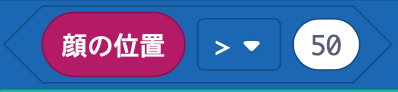What does this mean?
The position of the face in the camera is in the "Face position" block.
And if it is greater than 50, it is correct.

In other words, if you make a "if" block, it can see that the face is on the right side!
This is OK.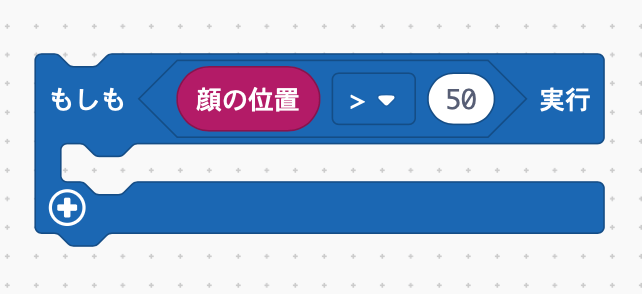Let's add it not only on the right but also on the left.
You press the plus at the bottom left of if block,But this time the condition that Tara less than -50.And prepare "other" which is neither.The other is that the face is roughly in the middle of -50 to 50.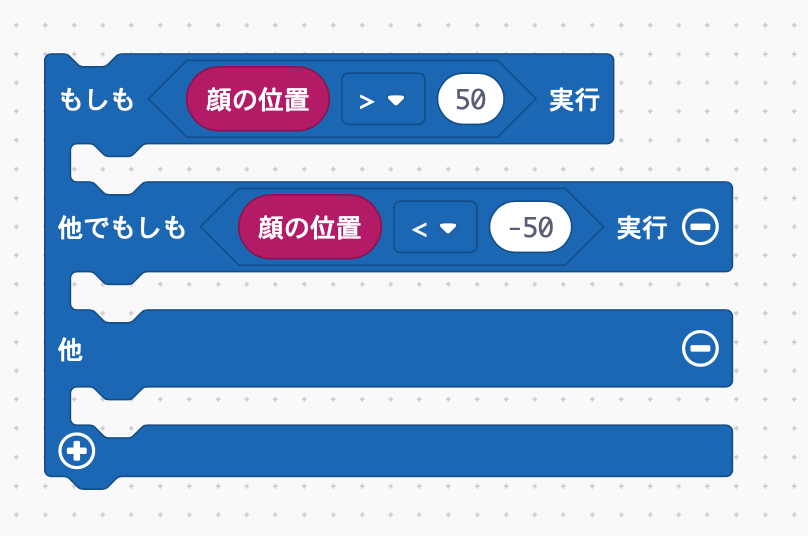## Motor output change

Assuming that the camera shows that there are people on the right or left
How can motor slowly turn to the right or left instead of straight or rotating?

There is a block called "motor rotation".
In this block you can change the power of the motor using numbers.

And we can set different values for left and right.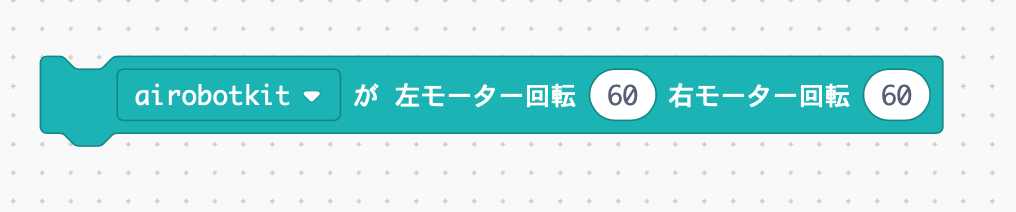If we use this, you can make only the right motor a little weaker.
Then it will slowly move to the right.

Let's use this to weaken the right motor a little and move it to the right when your face is to the right, and weaken the left when it is to the left.

And if neither is the case, try turning the motor with the same power.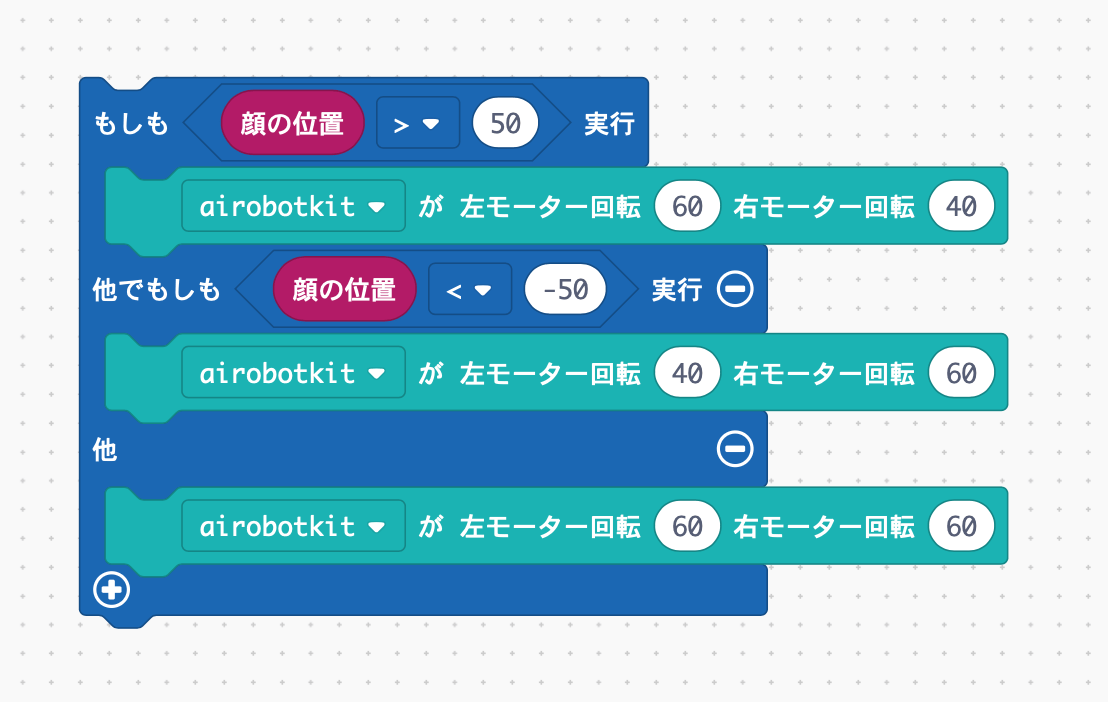It looks like this.

Come on! done!
That is completed we put this if block in the "If the face is in the camera"!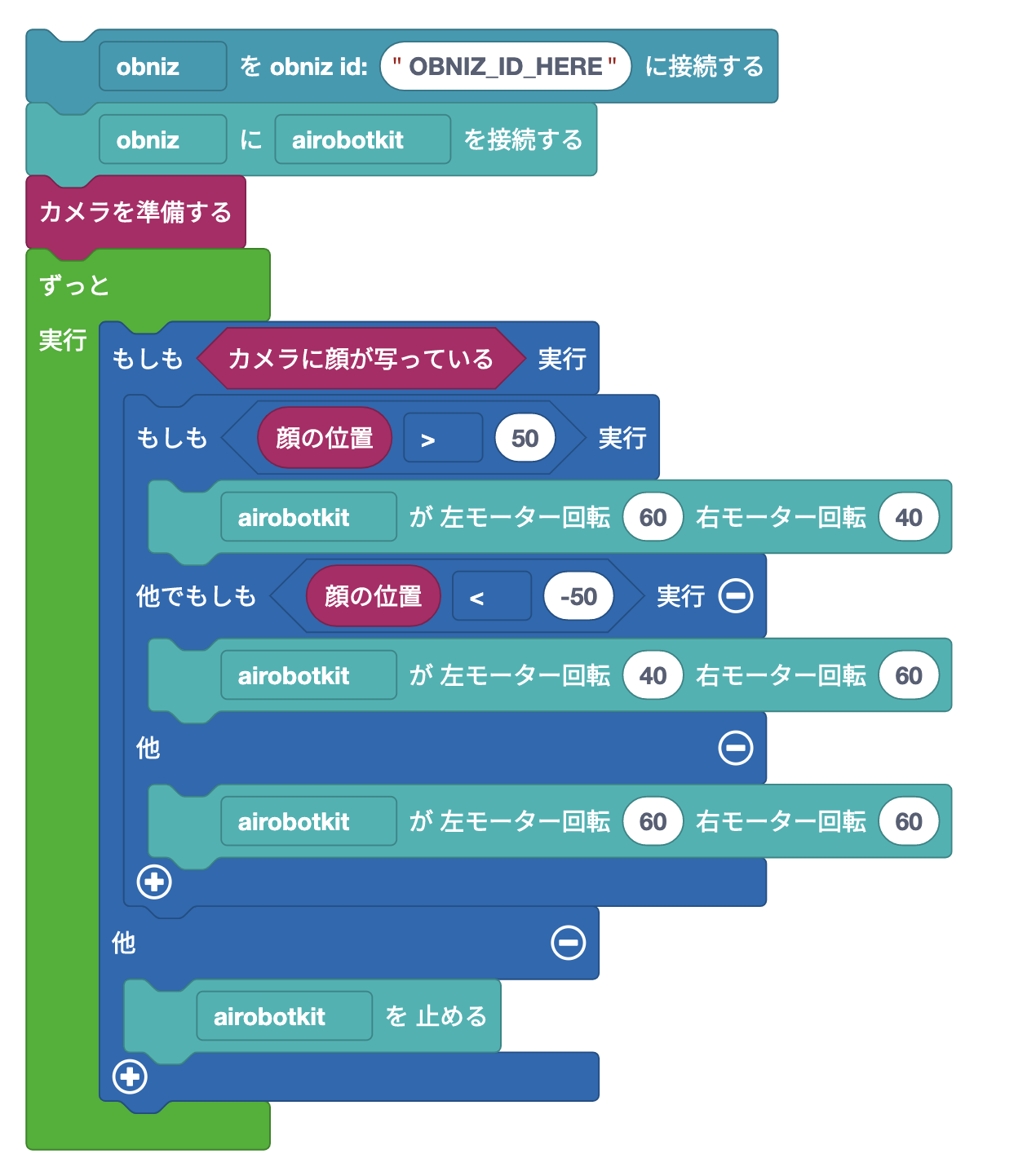Let's move it.